# Ratio Analysis | Accounts Class 12

When one number is expressed in terms of other, it is known as ratio. A ratio analysis is a quantitative analysis of information contained in a company’s financial statements.

The ratios may be categorised into the following four types:

1. Pure Ratio or Simple Ratio: It is obtained by dividing one number by another. It is the simplest form of ratio.
2. Percentage : In this type of ratios, the relationship between two number is expressed in hundredth or percentage.
3. Fraction : In this type of ratios, the relationship between two number is expressed in fractions.
4. Rate : In this type of ratios, a comparison is made between two numbers in ‘times’, i.e. one number is how many times the other number.

Accounting ratios are used to analyse the financial position of the firm. They are being categorised into different types which are given as below:

1. Balance Sheet Ratios
2. Statement of Profit & Loss Ratios
3. Composite Ratios

### Calculation of current assets turnover ratio

#### Ratio Analysis Example 1:

From the following information calculate the Current assets turnover ratio: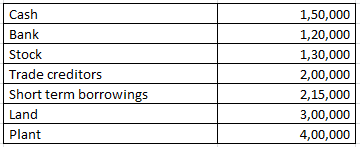Revenue from the operation for the year were Rs. 2800000

Explanation : –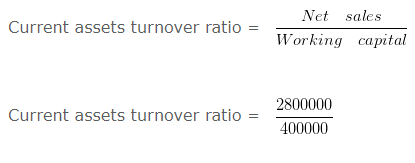Current assets turnover ratio = 7 Times
Working note 1 : Current assets = Cash + Bank + Stock
Current assets = 150000 + 120000 + 130000
Current assets = 400000

### Calculation of inventories when Inventory Ratio is given

#### Ratio Analysis Example 2:

Calculate the value of opening Inventory from the following information.
Cost of revenue from operations is 1200000 and Inventory turnover ratio is 3 Times. and opening inventory is 40000 less than the closing inventory

Explanation : –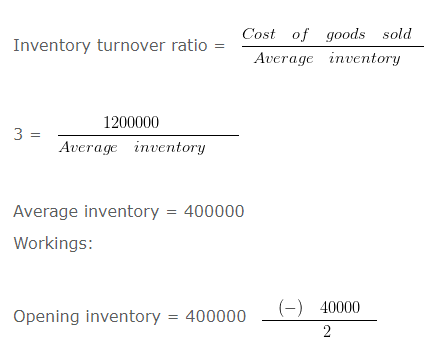Opening inventory 380000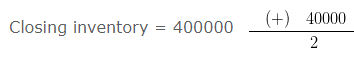Closing inventory = 420000

#### Ratio Analysis Example 3:

Calculate the value of opening Inventory from the following information:
Cost of revenue from operations is 16000 and Inventory turnover ratio is 1 Times. and opening inventory is 6 Times More than the closing inventory.

Explanation : –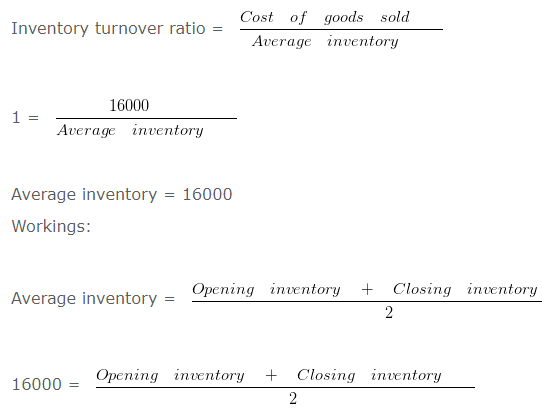32000 = Opening inventory + Closing inventory

Since the opening inventory is 6 Times More than the closing inventory therefore the ratio between opening inventory and closing inventory will be 7 : 1

Opening inventory = 32000 X 7/8

Opening inventory = 28000

Closing inventory = 32000 X 1/8

Closing inventory = 4000

### Computation of current ratio

#### Ratio Analysis Example 4: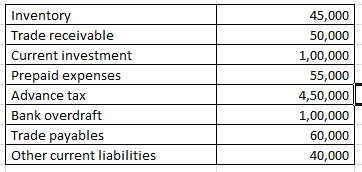On the basis of the following information calculate the current ratio:

Explanation : –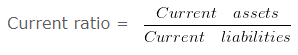Current ratio = 700000/200000

Current ratio = 3.5 :1
Working note 1 : Current assets = Inventory + Trade receivable + Current investment + Prepaid expenses + Advance tax
Current assets = 45000 + 50000 + 100000 + 55000 + 450000
Current assets = 700000
Working note 2 : Current liabilities = Bank overdraft + Trade payables + Other current liabilities
Current liabilities = 100000 + 60000 + 40000
200000

### Computation of debt equity ratio

#### Ratio Analysis Example 5:

From the following information calculate the debt equity ratio.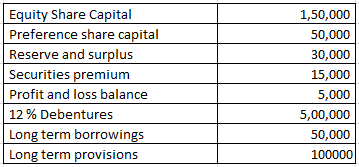Explanation : –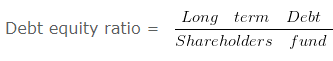Debt equity ratio = 650000/250000

Debt equity ratio = 2.6 : 1
Working note 1 : Long term Debt = 12 % Debentures + Long term borrowings + Long term provisions
Long term Debt = 500000 + 50000 + 100000
Long term Debt = 650000
Working note 2 : Shareholders fund = Equity Share Capital + Preference share capital + Reserve and surplus + Securities premium + Profit and loss balance
Shareholders fund = 150000 + 50000 + 30000 + 15000 + 5000
Shareholders fund = 250000

### Computation of liquidity ratio

#### Ratio Analysis Example 6:

On the basis of the following information calculate the liquidity ratio: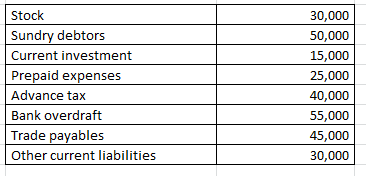Explanation : –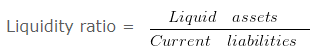Liquidity ratio = 65000/130000

Liquidity ratio = 0.5 :1
Working note 1 : Liquid assets = Current assets (-) ( Stock + Prepaid expenses + Advance tax )
Liquid assets = 160000 (-) 30000 + 25000 + 40000
Liquid assets = 65000
Working note 2 : Current assets = Stock + Sundry debtors + Current investment + Prepaid expenses + Advance tax
Current assets = 30000 + 50000 + 15000 + 25000 + 40000
Current assets = 160000
Working note 3 : Current liabilities = Bank overdraft + Trade payables + Other current liabilities
Current liabilities = 55000 + 45000 + 30000
130000

### Computation of quick ratio

#### Ratio Analysis Example 7:

On the basis of the following information calculate the Quick ratio: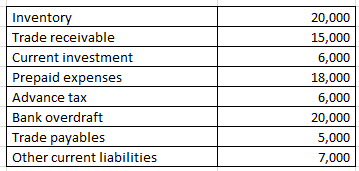Explanation : –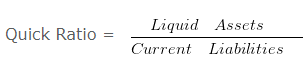Quick ratio = 21000/12000

Quick ratio = 1.75 :1
Working note 1 : Liquid assets = Current assets (-) ( Inventory + Prepaid expenses + Advance tax )
Liquid assets = 65000 (-) 20000 + 18000 + 6000
Liquid assets = 21000
Working note 2 : Quick liabilities = Current liabilities (-) Bank overdraft
Quick liabilities 32000 (-) 20000
Quick liabilities 12000
Working note 3 : Current assets = Inventory + Trade receivable + Current investment + Prepaid expenses + Advance tax
Current assets = 20000 + 15000 + 6000 + 18000 + 6000
Current assets = 65000
Working note 4 : Current liabilities = Bank overdraft + Trade payables + Other current liabilities
Current liabilities = 20000 + 5000 + 7000
32000

### when current liabilities are given

#### Ratio Analysis Example 8:

From the following information compute the current ratio.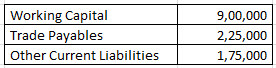Explanation : –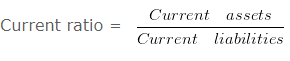Current Ratio = 1300000/400000

Current Ratio = 3.25 :1
Workings:
Working Capital = Current assets (-) current liabilities
900000 = Current assets (-) 400000
Current assets = 1300000
Workings:
current liabilities = Trade Payables (+) Other Current Liabilities
current liabilities = 225000 (+) 175000
current liabilities = 400000

### when total debts are given

#### Ratio Analysis Example 9:

From the following information compute the current ratio.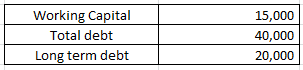Explanation : –Current Ratio = 35000/20000

Current Ratio = 1.75 :1
Workings:
Working Capital = Current assets (-) Current liabilities
15000 = Current assets (-) 20000
Current assets = 35000
Workings:
Current liabilities = Total debt (-) Long term debt
Current liabilities = 40000 (-) 20000
Current liabilities = 20000

### when inventory and current assets given

#### Ratio Analysis Example 10:

From the following information compute the current ratio.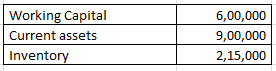Explanation : –Current Ratio = 900000/300000

Current Ratio = 3 :1
Workings:
Working Capital = Current assets (-) Current liabilities
600000 = 900000 (-) Current liabilities
Current liabilities = 300000

### Debt equity ratio

#### Ratio Analysis Example 11:

From the following information calculate debt equity ratio.

Explanation : –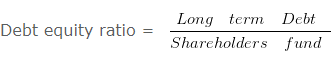Debt equity ratio = 200000/400000

Debt equity ratio = 0.5 : 1
Working note 1 : Long term Debt = Long term borrowings + Long term provisions
Long term Debt = 120000 + 80000
Long term Debt = 200000
Working note 2 : Shareholders fund = Non current assets + Working capital (-) Non current liabilities
OR
Shareholders fund = Non current assets + Current assets (-) Current liabilities (-) Long term borrowings (-) Long term provisions
Shareholders fund = 500000 + 200000 (-) 100000 (-) 120000 (-) 80000
Shareholders fund = 400000

### Determination of working capital

#### Ratio Analysis Example 12:

From the following given information:
A firm had current assets of RS. 140000
it then paid a current liability of RS. 20000
After this payment the current ratio was 3 : 2
Determine the current liabilities and working capital after the payment.

Explanation : –

Working capital = Current assets (-) Current liabilities
= 120000 (-) 80000
= 40000
Workings:
Current Assets = 140000
Current Assets After the payment of RS. 20000 would be 140000 (-) 20000 = 120000
As current ratio is 3 : 2 and current assets are RS. 1200003/2 = 120000/Current liabilities

Current liabilities = 240000/3

Current liabilities = 80000

### Determination of working capital when current ratio given

#### Ratio Analysis Example 13:

From the following information:
A firm had current assets of RS. 225000
it then paid a current liability of RS. 45000
After this payment the current ratio was 9 : 7
Determine the current liabilities and working capital Before the payment.

Explanation : –

(i)
Working capital = Current assets (-) Current liabilities
= 180000 (-) 140000
= 40000
Workings:
Current Assets = 225000
Current Assets After the payment of RS. 45000 would be 225000 (-) 45000 = 180000
As current ratio is 9 : 7 and current assets are RS. 1800009/7 = 180000/Current liabilities

Current liabilities = 1260000/9

Current liabilities = 140000
Before the payment of liabilities of RS. 45000 total amount of
Current liabilities = 45000 + 140000
Current liabilities = 185000
Working capital = 40000

### Fixed assets turnover ratio

#### Ratio Analysis Example 14:

From the following information, calculate fixed assets turnover ratio:

Revenue from the operation for the year were RS. 2000000

Explanation : –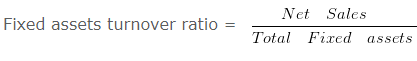Fixed assets turnover ratio = 2000000/500000

Fixed assets turnover ratio = 4 Times
Total Fixed assets = Land + Building + Furniture
Total Fixed assets = 300000 + 50000 + 150000
Total Fixed assets = 500000

### Inventory turnover ratio

#### Ratio Analysis Example 15:

Calculate Inventory turn over ratio from the following information.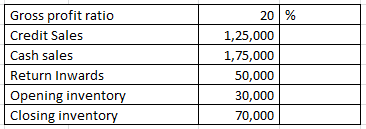Explanation : –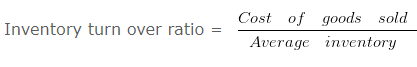Inventory turn over ratio = 200000/50000

Inventory turn over ratio = 4 Times
Workings:
Cost of goods sold = Net Sales (-) gross profit
250000 (-) 20% X 250000
250000 (-) 50000
200000
Net Sales = Cash sales + Credit Sales – Return Inwards
175000 + 125000 – 50000
250000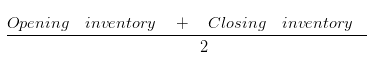= (30000+70000)/2

= 50000

### Inventory turnover ratio and average age of inventory

#### Ratio Analysis Example 16:

Calculate Inventory turnover ratio and average age of inventory from the following information: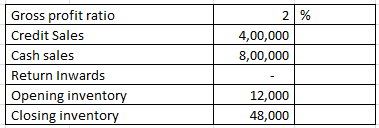Explanation : –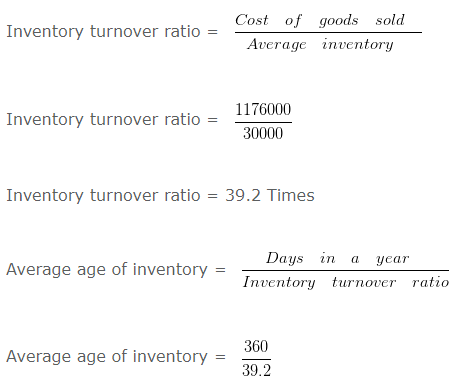= 9.18 Days
Workings:
Cost of goods sold = Net Sales (-) gross profit
1200000 (-) 2% X 1200000
1200000 (-) 24000
1176000
Net sales = Cash sales + Credit Sales (-) Return Inwards
800000 + 400000 (-) 0
1200000

Average inventory

== (12000+48000)/2

= 30000

### Inventory turnover ratio when closing stock is given

#### Ratio Analysis Example 17:

Calculate Inventory turnover ratio from the following information: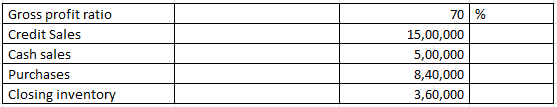Explanation : –Inventory turn over ratio = 600000/240000

= 2.5 Times
Workings:
Cost of goods sold = Net Sales (-) gross profit
= 2000000 (-) 70% x 2000000
= 2000000 (-) 1400000
= 600000
Net sales = Cash sales + Credit Sales
500000 + 1500000
2000000

Average inventory

== (120000+360000)/2

Average inventory = 240000
Cost of goods sold = Opening inventory + Purchases (-) Closing inventory
600000 = Opening inventory + 840000 (-) 360000
= Opening inventory + 480000
Opening inventory = 120000

### Inventory turnover ratio when GP ratio based on cost

#### Ratio Analysis Example 18:

Calculate Inventory turn over ratio from the following information: Gross profit ratio is 10 % Of cost and revenue from operation is RS. 770000 . Opening inventory was 9/11 of closing inventory and closing inventory was 10 % of revenue from operation.

Explanation : –Inventory turnover ratio = 700000/70000

Inventory turnover ratio = 10 Times
Working note: = Gross profit ratio is 10% Of cost
Therefore goods costing Rs. = 100 is sold for RS. 110
Cost of goods sold = 100
If revenue from operation is = 770000

Cost of goods sold = 700000 ( 770000 X 100/110 )

Average inventory

== (63000+77000)/2

= 70000
Closing inventory = 10% x Revenue from operation
Closing inventory = 10% x 770000
Closing inventory = 77000

Opening inventory = 9/11 x Closing inventory

Opening inventory = 9/11 x 77000

Opening inventory = 63000

### Inventory turnover ratio when GP ratio is negative

#### Ratio Analysis Example 19:

Calculate Inventory turnover ratio from the following information: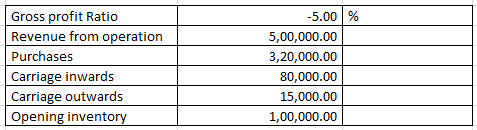Explanation : –Inventory turnover ratio = 525000/37500

Inventory turnover ratio = 14 Times
Workings:
Cost of goods sold = Revenue from operation (-) gross profit
= 500000 (-) -5% X 500000
= 500000 (-) -25000
= 525000

Average inventory

== [100000+(-25000)]/2

= 37500

### Inventory turnover ratio when opening stock is given

#### Ratio Analysis Example 20:

Calculate Inventory turn over ratio from the following information: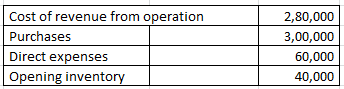Explanation : –Inventory turn over ratio = 280000/80000

Inventory turn over ratio = 3.5 Times
Workings:
Cost of goods sold = Opening inventory + Purchases + Direct expenses – Closing inventory
280000 = 40000 + 300000 + 60000 – Closing inventory
Closing inventory = 120000

Average inventory

== (40000+120000)/2

Average inventory = 80000

### Total assets turnover ratio

#### Ratio Analysis Example 21:

From the following information calculate the total assets turnover ratio.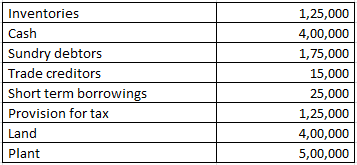Revenue from the operation for the year were RS. 4800000

Explanation : –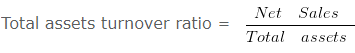Total assets turnover ratio = 4800000/1600000

Total assets turnover ratio = 3 Times
Working note 1 : Total assets = Inventories + Cash + Sundry debtors + Land + Plant
Total assets = 125000 + 400000 + 175000 + 400000 + 500000
Total assets = 1600000

### Working capital turnover ratio

#### Ratio Analysis Example 22:

From the following information calculate the working capital turnover ratio: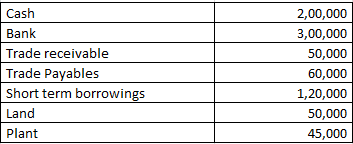Revenue from the operation for the year were RS. 1850000

Explanation : –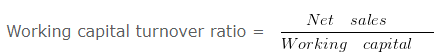Working capital turnover ratio = 1850000/370000

Working capital turnover ratio = 5 Times
Working note 1 : Working capital = Current assets (-) Current liabilities
Working capital = 550000 (-) 180000
Working capital = 370000
Working note 2 : Current assets = Cash + Bank + Trade receivable
Current assets = 200000 + 300000 + 50000
Current assets = 550000
Working note 3 : Current liabilities = Trade Payables + Short term borrowings
Current liabilities = 60000 + 120000
Current liabilities = 180000

### Liquid ratio on the basis of working capital

#### Ratio Analysis Example 23:

Current Assets = Rs. 80000 . Inventory = Rs. 15000 . Prepaid Expenses = Rs. 50000 . Working Capital = Rs. 50000 Calculate Liquid Ratio.

Explanation : –

Liquid Assets = Current Assets (-) Inventory (-) Prepaid Expenses
Liquid Assets = 80000 (-) 15000 (-) 50000
Liquid Assets = 15000
Current Liabilities = 30000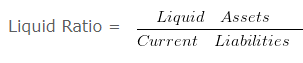Liquid Ratio = 15000/30000

Liquid Ratio = 0.5
Working Note 1 :
Current Liabilities = Current Assets (-) Working Capital
= 80000 (-) 50000
= 30000

### Calculation of capital employed

#### Ratio Analysis Example 24:

Calculate return on capital employed from the following information.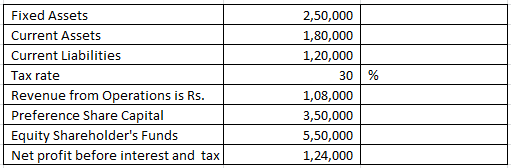Explanation : –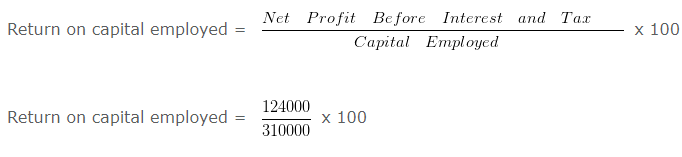Return on capital employed = 40 %
Working note 1 :
Capital employed = Fixed Assets + Current Assets (-) Current Liabilities
Capital employed = 250000 + 180000 (-) 120000
Capital employed = 310000

### Calculation of Dividend Payout Ratio

#### Ratio Analysis Example 25:

On the basis of the following data calculate Dividend Payout Ratio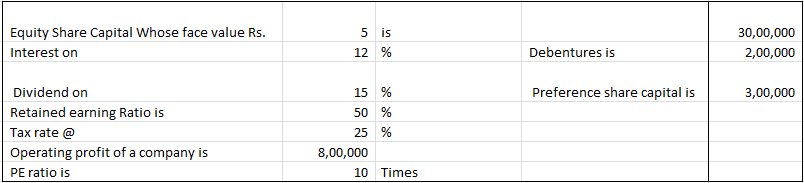Explanation : –

EBIT = 800000
Less: Interest on Debentures = 200000
EBT = 600000
Less: Tax = 150000
EAT = 450000
Less: Preference Dividend = 300000
Earnings for Equity Shareholders = 150000
Less: Retained Earnings = 75000
Dividend Paid = 75000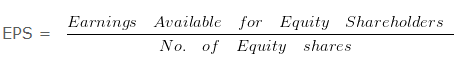EPS = 150000/600000

EPS = 0.25 Per share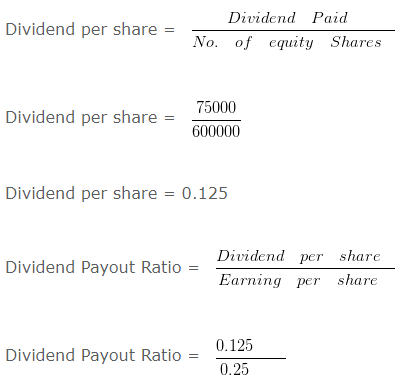Dividend Payout Ratio = 50 %

### Calculation of Dividend Per Share

#### Ratio Analysis Example 26:

On the basis of the following data calculate dividend per share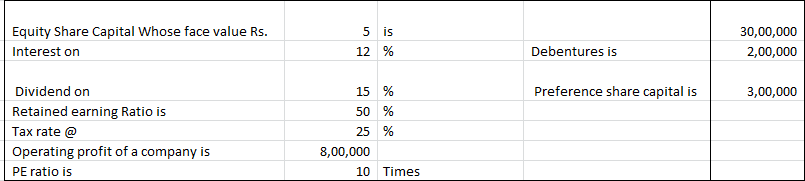Explanation : –

EBIT = 800000
Less: Interest on Debentures = 200000
EBT = 600000
Less: Tax = 150000
EAT = 450000
Less: Preference Dividend = 300000
Earnings for Equity Shareholders = 150000
Less: Retained Earnings = 75000
Dividend Paid = 75000EPS = 150000/600000

EPS = 0.25 Per share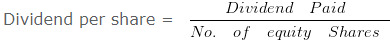Dividend per share = 75000/600000

Dividend per share = 0.125

### Calculation of Earning Per Share

#### Ratio Analysis Example 27:

On the basis of the following data calculate earning per share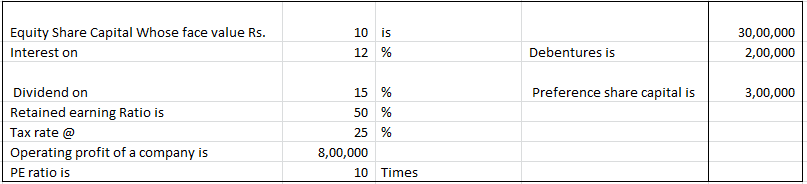Explanation : –

EBIT = 800000
Less: Interest on Debentures = 200000
EBT = 600000
Less: Tax = 150000
EAT = 450000
Less: Preference Dividend = 300000
Earnings for Equity Shareholders = 150000
Less: Retained Earnings = 75000
Dividend Paid = 75000EPS = 150000/300000

EPS = 0.5 Per share

### Calculation of Equity ratio

#### Ratio Analysis Example 28:

From the Following information calculate Equity Ratio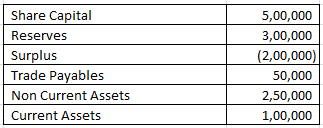Explanation : –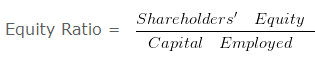Equity Ratio = 600000/300000

Equity Ratio = 2
Working note 1 :
Shareholders’ Equity = Share Capital + Reserves + Surplus
Shareholders’ Equity = 500000 + 300000 + -200000
Shareholders’ Equity = 600000
Capital employed = Non Current Assets + Current Assets (-) Trade Payables
Capital employed = 250000 + 100000 (-) 50000
Capital employed = 300000

### Calculation of Liquid Ratio

#### Ratio Analysis Example 29:

Current Assets of ABC Ltd. are Rs. 72000 and the current ratio is 1.2 . Value of inventories is Rs. 27000 . Calculate liquid ratio.

Explanation : –OR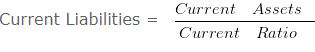Current Liabilities = 72000/1.2

Current Liabilities = 60000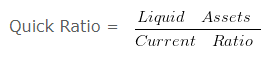Quick Ratio = 45000/60000

Quick Ratio = 0.75
Working Notes:
Liquid Assets = Current Assets (-) Inventory
Liquid Assets = 72000 (-) 27000
Liquid Assets = 45000

### Calculation of market price Per Share

#### Ratio Analysis Example 30:

On the basis of the following data calculate market price per share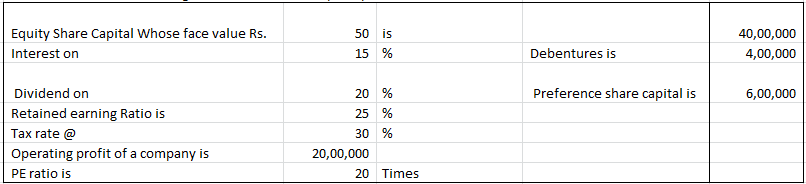Explanation : –

EBIT = 2000000
Less: Interest on Debentures = 200000
EBT = 1800000
Less: Tax = 720000
EAT = 1080000
Less: Preference Dividend = 200000
Earnings for Equity Shareholders = 880000
Less: Retained Earnings = 352000
Dividend Paid = 528000EPS = 880000/250000

EPS = 3.52 Per share
Market price per share = PE Ratio x EPS
Market price per share = 15 x 3.52
Market price per share = 52.8

### Calculation of Net working capital

#### Ratio Analysis Example 31:

Current Ratio is 2 : 1
Current Assets = 400000
Calculate Net Working Capital

Explanation : –

Net Working Capital = Current Assets (-) Current Liabilities
Net Working Capital = 400000 (-) 200000
Net Working Capital = 200000
Working note 1 := 200000

### Calculation of profit before interest and tax

#### Ratio Analysis Example 32:

Calculate profit before interest and tax from the following information.
Profit after interest and tax 1170000
15 % Debentures 300000
Tax @ 22 %

Explanation : –

Profit before interest and tax = Profit before tax + Interest
Profit before interest and tax = 1500000 + 45000
Profit before interest and tax = 1545000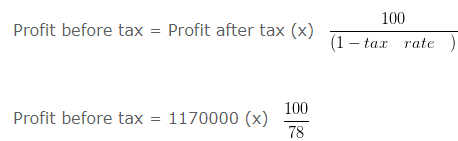Profit before tax = 1500000
Interest = Rate of interest (x) Debentures
Interest = 15% (x) 300000
Interest = 45000

### Calculation of Proprietary Ratio

#### Ratio Analysis Example 33:

Compute Proprietary ratio if equity share capital is Rs. 125000 ,Preference Share Capital is Rs. 100000 ,Capital Reserve is Rs. 80000 ,Profit & Loss Balance is Rs. 55000 . The value of 7 % Debentures is Rs. 62500 and 9 % Mortgage loan- Rs. 112500 .Value of Current Liabilities
is Rs. 262500 Non Current Assets is worth Rs. 275000 Value of Current Assets is Rs. 125000 .

Explanation : –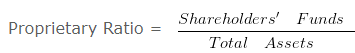Proprietary Ratio = 360000/400000

Proprietary Ratio = 0.9
Working note 1 : Shareholders’ Funds = Equity share capital + Preference share capital + Capital reserve + Profit and loss balance
Shareholders’ Funds = 125000 + 100000 + 80000 + 55000 Shareholders’ Funds = 360000
Working note 2 : Total Assets = Non current assets + Current assets
Total Assets = 275000 + 125000
Total Assets = 400000

### Capital Gearing Ratio

#### Ratio Analysis Example 34:

Compute Capital Gearing Ratio from the following information.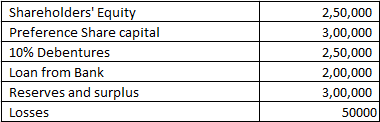Explanation : –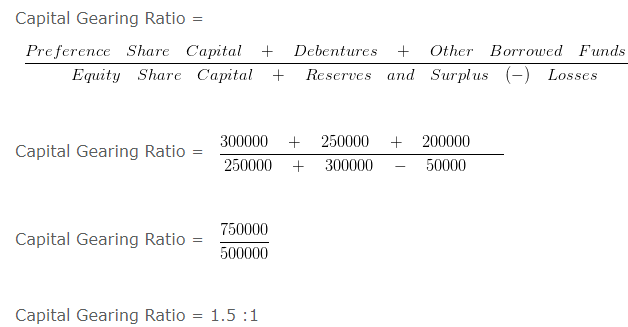### Current assets on the basis of deemed current ratio

#### Ratio Analysis Example 35:

The ratio of Current Assets (Rs. 225000 ) to Current Liabilities is 2.25 : 2 .
The firm is interested in maintaining a Current ratio of 1.5 : 1 by acquiring some Current Assets on credit.
You are required to suggest the amount of Current Assets which must be acquired for this purpose.

Explanation : –ORCurrent Liabilities = 450000/2.25

Current Liabilities = 200000
Working note 1 : Let x be the amount of current assets acquire on credit
Current Ratio to be maintained= 1.5 : 1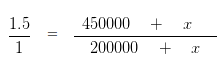300000 + 1.5x = 450000 + 1x
1.5x-1x = 150000
0.5x = 150000
x = 300000

### Current Ratio on the basis of Quick Ratio

#### Ratio Analysis Example 36:

Value of Inventory of HRD & Co. is Rs. 240000 . Liquid Assets are Rs. 120000
Quick Ratio is 0.5 . Calculate the current ratio .

Explanation : –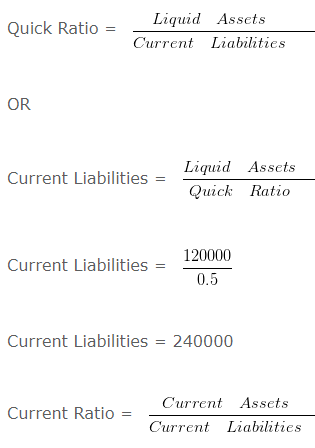Current Ratio = 360000/240000

Current Ratio = 1.5 :1
Working Notes:
Current Assets = Liquid Assets + Inventory
= 120000 + 240000
= 360000

### Debt to total assets ratio

#### Ratio Analysis Example 37: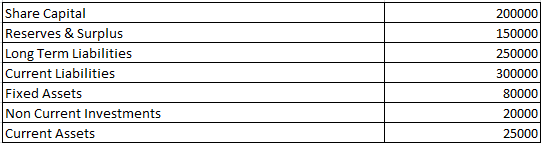Compute Debt to Total Assets Ratio from the above information.

Explanation : –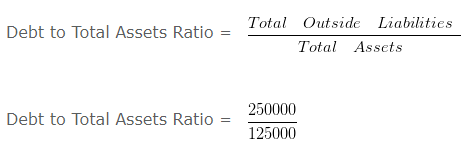Debt to Total Assets Ratio = 2 :1
Working note 1 :
Total Assets = Fixed Assets + Non Current Investments + Current Assets
Total Assets = 80000 + 20000 + 25000
Total Assets = 125000

### Determination of current assets when current ratio is given

#### Ratio Analysis Example 38:

Current Ratio of a business is 13 : 11 and Quick Ratio is 0.75 .
If Working Capital is Rs. 200000 then calculate the value of current assets and inventory.

Explanation : –

Working Capital = Current Assets (-) Current Liabilities
or
Current Liabilities = Current Assets (-) Working Capital
= 1300000 (-) 200000
= 1100000or
Liquid Assets = Current Liabilities x Quick Ratio
= 1100000 x 0.75
= 825000
Inventory = Current Assets (-) Liquid Assets
= 1300000 (-) 825000
= 475000
Working Note 1 :
Working Capital = Current Assets (-) Current Liabilities
Working Capital = 13 (-) 11
Working Capital = 2
When working capital = 2 then Current Assets = 13
When working capital = 200000 then Current Assets = 1300000

### Fixed Charges Coverage Ratio

#### Ratio Analysis Example 39:

Earnings before Interest & Taxes is Rs. 1000000 and Lease Payments is Rs. 200000 .Interest is Rs. 50000 .
Compute Fixed Charges Coverage Ratio.

Explanation : –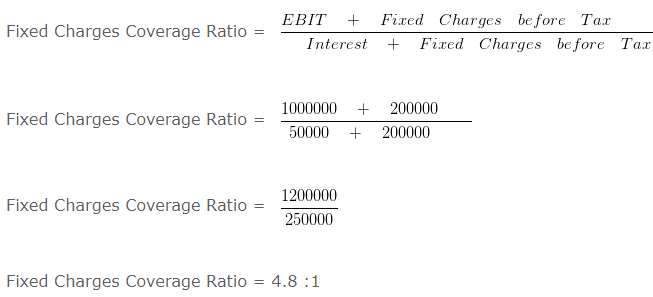### Gross Profit Ratio, Operating Ratio & Operating Profit Ratio

#### Ratio Analysis Example 40:

The following information is given
If Revenue from Operations of XYZ Ltd is Rs. 1000000 Cost of Revenue from Operations is Rs. 450000 Selling Expense is Rs. 80000 Administrative Expenses is Rs. 60000
Calculate- Gross Profit Ratio ,Operating Ratio , Operating Profit Ratio

Explanation : –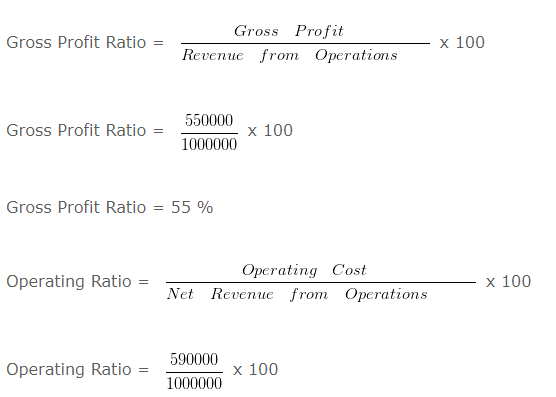Operating Ratio = 59 %
Operating Profit Ratio = 100 (-) Operating Ratio
Operating Profit Ratio = 100 (-) 59
Operating Profit Ratio = 41 %
Working Notes:
1. Gross Profit = Revenue from Operations (-) Cost of Revenue from Operations
1. Gross Profit = 1000000 (-) 450000
1. Gross Profit = 550000
2. Operating Cost = Cost of Revenue from Operations + Selling Expenses + Administrative Expenses
2. Operating Cost = 450000 + 80000 + 60000
2. Operating Cost = 590000

### Interest Coverage Ratio

#### Ratio Analysis Example 41:

Compute Interest Coverage ratio if equity share capital is Rs. 1200000 ,Preference Share Capital is Rs. 720000 ,Capital Reserve is Rs. 360000 ,Profit & Loss Balance is Rs. 600000 . The Value of 13 % debentures is Rs. 250000 and 11 % Mortgage loan of Rs. 300000 .The value of Current Liabilities is Rs. 1180000 Non Current Assets is worth Rs. 2400000 Value of Current Assets is Rs. 3000000 .

Explanation : –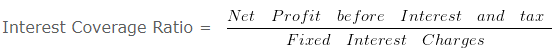Interest Coverage Ratio = 589500/65500

Interest Coverage Ratio = 9 times
Working Notes:
Interest on debenture = 13% x 250000
= 32500
Interest on loan = 11% x 300000
33000
Total interest charges = 65500

### Interest Coverage Ratio & Debt Service Coverage Ratio

#### Ratio Analysis Example 42:

Calculate- Interest Coverage Ratio & Debt Service Coverage Ratio from the following information. Net Profit before interest and tax is Rs. 300000 . 5 % Long Term Debt 500000 (Principle amount is repayable in 10 equal installments) .

Explanation : –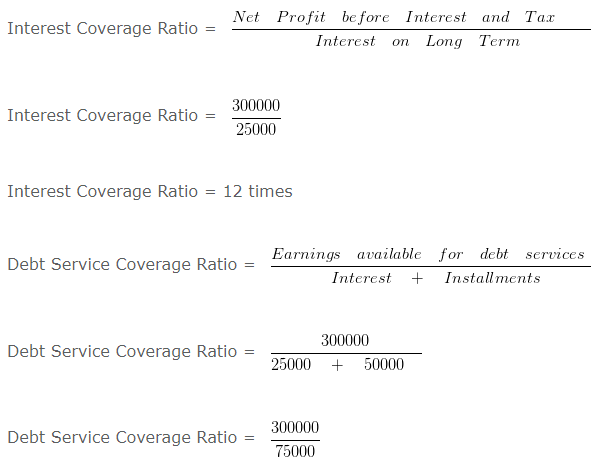Debt Service Coverage Ratio = 4 times
Working Notes:
1. Interest on Long Term Debt = 5 % x 500000
25000

### Return on Assets

#### Ratio Analysis Example 43:

From the following information calculate return on total assets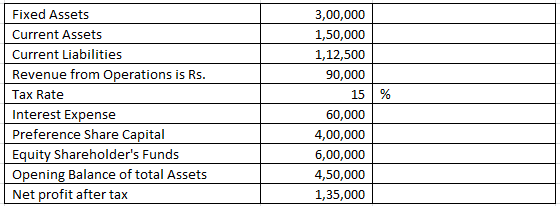Explanation : –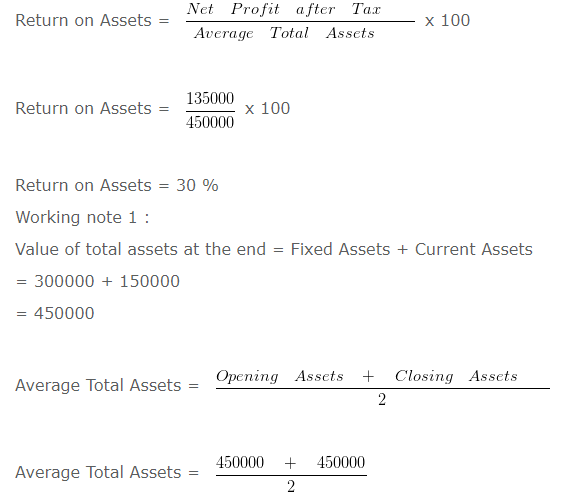Average Total Assets = 450000

### Return on Assets & Return on Capital Employed

#### Ratio Analysis Example 44:

Calculate Return on Assets & Return on Capital Employed on the basis of the following information.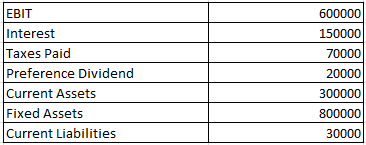Explanation : –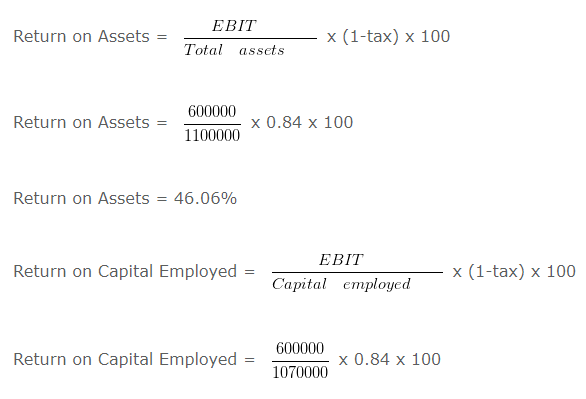Return on Capital Employed = 47.35%
Working Notes:
EBT = EBIT (-) Interest
EBT = 600000 (-) 150000
EBT = 450000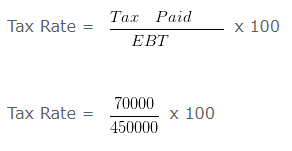Tax Rate = 15.56 %
Tax Rate = 15.56%
2. Total Assets = 1100000
3. Capital Employed = 1070000

### Return On Equity

#### Ratio Analysis Example 45:

Calculate return on equity from the following information.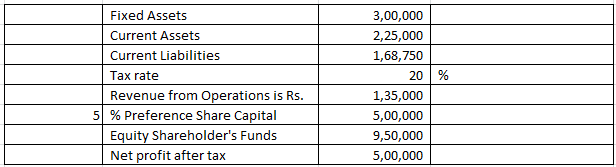Explanation : –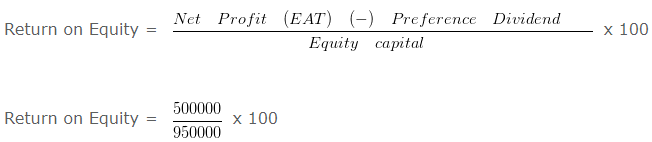Return on Equity = 50 %

### Trade payable turnover ratio

#### Ratio Analysis Example 46:

Calculate the trade payable turnover ratio and average payment period From the following information. Credit purchases during the 2016-2017 is 1000000 . Balance of opening Creditors and Bills payable on 01.04.2016 is RS. 40000 and 15000 and the Balance of Closing Creditors and bills payable on 31.03.2017 is RS. 35000 and 10000

Explanation : –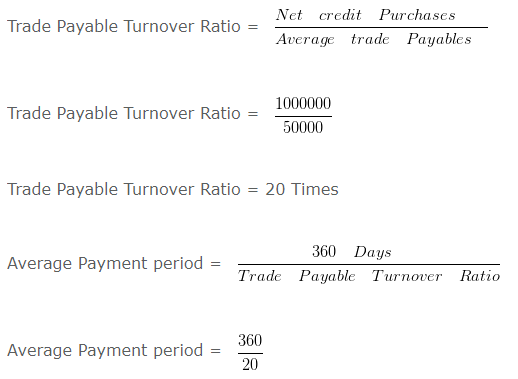Average Payment period = 18 Days
Workings: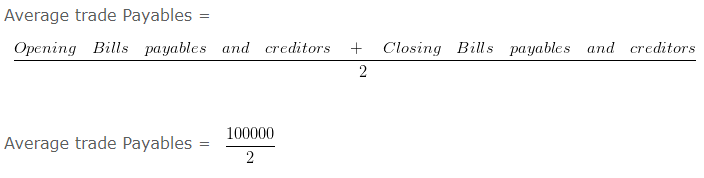Average trade Payables = 50000

### Trade receivable turnover ratio

#### Ratio Analysis Example 47:

Calculate the Trade receivable Turnover Ratio and average collection period from the following information: Total revenue from the operation is 100000 and cash revenue is 10 % of total revenue from the operation. Balance of opening receivable on 01.04.2016 is RS. 60000 and the Balance of Closing receivable on 31.03.2017 is 40000

Explanation : –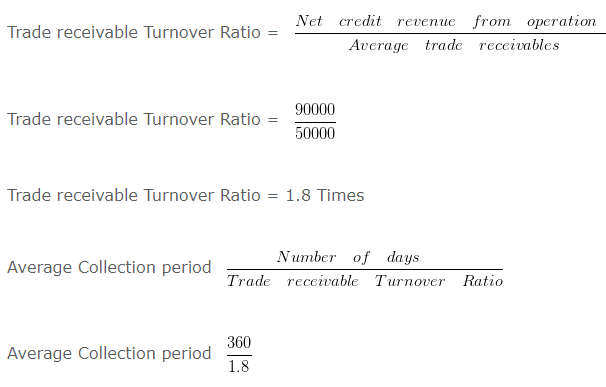Average Collection period = 200 Days
Workings:
Credit sales = 100000 x 0.9
= 90000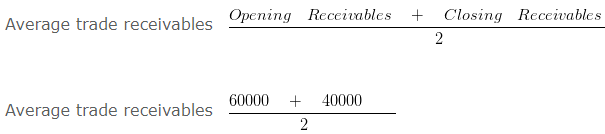Average trade receivables 50000